## Saturday, January 27, 2018

### Ground Contact Variables Affect External Running Power Derived From Accelerometry

In my previous post, I reviewed Stryd's running power model. While looking into Stryd's whitepaper and reading several other journal papers, I suspected that if running powermeter algorithms employed summation of energy changes at center of mass, then could variation in detected ground contact variables explain some of the striking variations in reported power between competing accelerometer platforms in the market?

Ground contact variables are things like ground contact force, ground contact time, stance time, step time, step rate, vertical oscillation and so on. Minimizing errors between detected variables and laboratory equipment may minimize variations in computed power, however we are yet to understand how these accelerometers work in outside running relative to variety of footstrikes and terrain types and how those errors vary relative to actual variables.

To understand what the effect of ground contact variables is on computed running power, I played around with some hard numbers and did a sensitivity analysis using a power model I built.

First, I got in touch with Professor Alberto Minetti to get some raw force plate data for running. Professor Minetti is widely regarded as an expert in the biomechanics of running and is an honorary research professor at Accademia Nazionale dei Lincei in Rome, Italy. He also leads the Laboratory of Physiomechanics of Locomotion at the Department of Pathophysiology and Transplantation at the University of Milan.

Prof. Minetti shared force plate data for a shod front foot striker running at 4 m/s, which is 14.4 kph or 6:42 min/mile.

I then developed a model to estimate the rate of changes in external work done using the EESA algorithm (see previous post). The model applies the same computation algorithm for external power as described in several sources in literature.

I. Effect of Variation in Detected Ground Contact Time On Running Power

Stryd's whitepaper showed that the error in ground contact time between force plate and their footpod IMU is 2.83%. The running speed and footstrike type within the data was not discussed. These images from my previous post are reproduced here.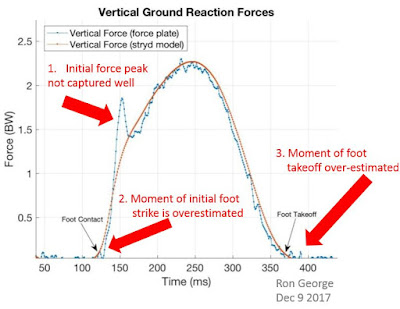Figure 1 : Modeled vs actual vertical force-time signatures in a rear-footed runner for an unspecified running speed. Base image courtesy of Stryd. Markups by the me. Observe that the Stryd thinks the footstrike is front footed when the force-time signature from the forceplate shows a rear foot strike.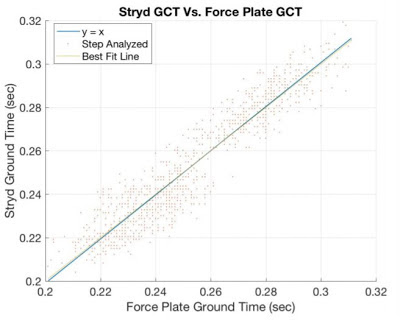Figure 2 : Plot showing goodness-of-fit of modeled GCT to force plate measurements. The average error is stated to be 2.83%. The number of runners, running speeds, shoes worn, footstrike mechanics and slope on the treadmill are all unknown which raises the question of how the error varies as a function of each of those factors.  Image courtesy of Stryd.

Now to the data from Prof. Minetti and my model :

Time signatures of the forces in the front foot striking runner are shown in Figure 3, where Fx, Fy and Fz are antero-posterial (A-P, or horizontal), vertical and medio-lateral forces respectively. Mechanical energy changes for all steps in the data and per step are shown in Figures 4 & 5 respectively.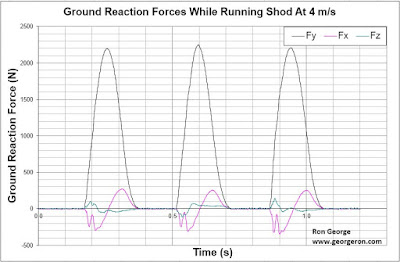Figure 3 : Force-time signatures for a 67kg front foot striking shod runner running at 4 m/s. Force plate data of Minetti, Milan (Italy). Force plate acquisition frequency = 1000 Hz.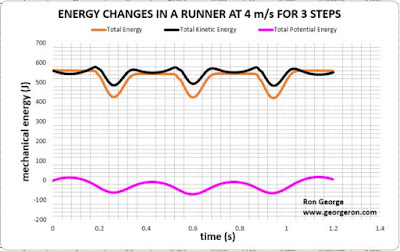Figure 4 : Mechanical energy changes for a 67kg front foot striking shod runner running at 4 m/s for 3 steps.Figure 5 : Mechanical energy changes for a 67kg front foot striking shod runner running at 4 m/s for a single step.

External power (Pext), vertical power (Pv), horizontal power (Px) and lateral power (Pz) are shown in the tabulated data in Figure 6 for a -5 to +5% variation in detected ground contact times.Figure 6 : External running power and it's 3D components per step for a rear footed shod runner running at 4 m/s. Values were computed using the EESA algorithm (or Cavagna method) as described in literature for a range of ground contact times from -5% to +5% relative to the highlighted base value. Computer model was developed by Ron George.

As shown in Figure 6, a variation of -5 to +5% in detected ground contact time has an impact of -5 to +5% in estimated external running power, all other factors kept the same.

Here, you can see power extending from 365 Watts to 331 Watts because of the error in vertical force-time signatures.

A computational difference between platforms for ground contact time can arise from 3
factors :

1. Errors in estimated ground force-time signatures from accelerometry.
2. Inability to differentiate between rear foot strike and front foot strike and suble variations in between.
3. Variations in the minimum force threshold set in the power algorithm while computing ground contact time. In literature, the threshold value from a minimum of 10 N to as large as 50 N, which translates to an appreciable difference in estimated stance times for a given running speed.

This is just one source of variation possible between different accelerometers in reported power. Another example of variation is from differences in estimated vertical oscillation or vertical translation of the center of mass.

II. Effect of Variation in Detected Vertical Oscillation On Running Power

The Stryd whitepaper revealed that an error of 3.18% existed between force plate vertical oscillation and that derived from the footpod. This is shown in Figure 7.Figure 7 : Estmated vs actual vertical oscillation in the Stryd footpod. Stated average error with respect to force plate = 3.18%. Base image courtesy of Stryd. Markups by me.

What does an error of 3.18% in vertical oscillation mean? It simply means that there is a difference in the vertical landing force that the footpod detects relative to force plate data. Since vertical velocity and vertical oscillation are single and double integrals of detected vertical accelarations respecitvely,  any error in the acceleration signal translates into errors in the vertical oscillation.

Now to the data from Prof. Minetti and my model :

External power (Pext), vertical power (Pv), horizontal power (Px) and lateral power (Pz) are shown in the tabulated data in Figure 8 for a -5 to +5% variation in accelerometer derived vertical oscillations.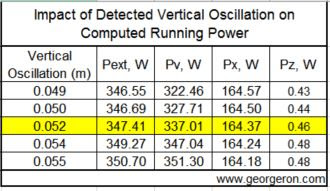Figure 8 : External running power and it's 3D components per step for a front footed shod runner running at 4 m/s. Values were computed using the EESA algorithm (or Cavagna method) as described in literature for a range of vertical oscillations from -5% to +5% relative to the highlighted base value. Computer model was developed by Ron George.

As shown in Figure 8, a variation of -5 to +5% in derived vertical oscillation has an impact of -0.3 to +1% in estimated external running power respectively, all other factors kept the same.

Here, you can see power extending from 347 Watts to 351 Watts because of the error in vertical force-time signatures.

A computational difference between platforms for vertical oscillation can arise from errors in the vertical acceleration and vertical force-time signatures computed by the device.

III. Effect of Variation in Horizontal Speed On Running Power

The Stryd whitepaper revealed that an error of 5% existed between force plate horizontal speed and that derived from the footpod. This is shown in Figure 9.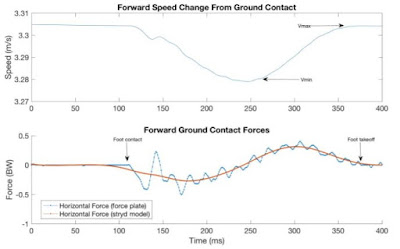Figure 9 :  Stryd modeled forward speed change compared to force plate measures. Indicated accuracy = 95%. Grade of running surface unknown, but presumably level. Image courtesy of Stryd.

Now to the data from Prof. Minetti and my model :

External power (Pext), vertical power (Pv), horizontal power (Px) and lateral power (Pz) are shown in the tabulated data in Figure 10 for a -5 to +5% variation in accelerometer derived horizontal speed.Figue 10 : External running power and it's 3D components per step for a front footed shod runner running at 4 m/s. Values were computed using the EESA algorithm (or Cavagna method) as described in literature for a range of horizontal speeds from -5% to +5% relative to the highlighted base value. Computer model was developed by Ron George.

As shown in Figure 10, a variation of -5 to +5% in derived horizontal speed has an impact of -7 to +16% in estimated external running power respectively, all other factors kept the same.

Here, you can see power extending from 324 Watts to 402 Watts because of the error in horizontal force-time signatures.

This analysis is a little incomplete because if horizontal speed changes, so can the vertical force-time signature. Therefore, there are multiplicative effects on computed power from the coupling of horizontal speed and vertical force.

A computational difference between platforms for horizontal speed can arise from errors in the horizontal acceleration and horizontal force-time signatures computed by the device.

CONCLUSION

A running powermeter that utilizes ground contact variables in it's calculation of running power can spell out a range of values for power depending on variation in those ground contact inputs.

The impact of error in three of these ground contact variables, chiefly vertical oscillation, ground contact time and horizontal speed, were explored in this article by independantly varying force time signatures and inspecting their impact on computed power.

Other sources of error exist. For example, if an accelerometer and the algorithm used cannot distinguish between a front footed strike and a rear footed one, the underlying force impulse characteristic is misjudged. Misjudging impact force-time signature impacts the computed potental energy change and hence potential work.

Based on the analysis for one front foot runner at 4 m/s, one observes that some of the striking differences in reported power between accelerometer platforms like Stryd and Garmin may lie in the variations in derived ground contact variables. Out of the three explored variables, errors in vertical and horizontal force time signatures can make an appreciable impact on the theoretically computed power (See Figures 6 & 10).

It is my hope that this writeup gives runners and coaches a qualitative and quantitative feel for the impact of accelerometer error upon the new metric of running power. Basing training prescription on faulty devices and secondary & tertiary metrics derived from them can carry a risk. Not being aware of such risk within new technology has implications for both undertraining and over-reaching.